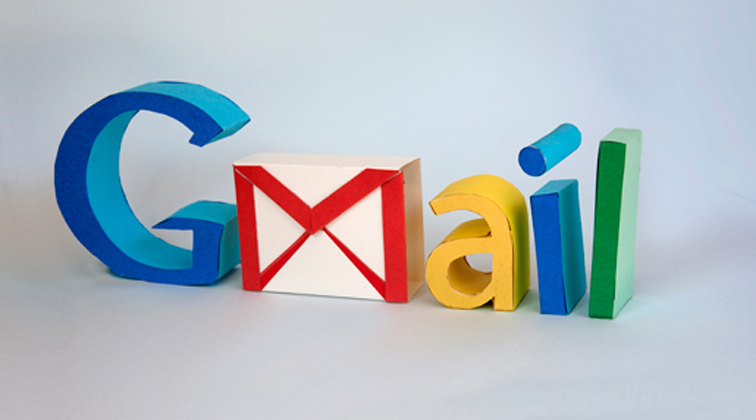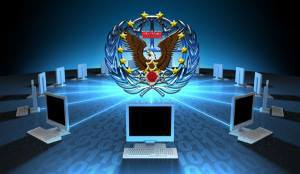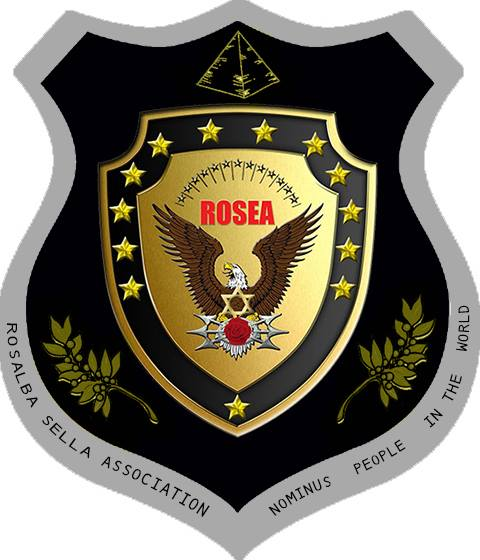RO.SE.A. Impressum:roseanpw@gmail.com

SITE ROSEA

www.rosea.eu

Für alle Ihre Fragen.

Diese Meldung wird absolut privat sein und nur von Rosalba SELLA lesen.)PRIVATER KONTAKTFORMULAR ( = 3 =)

Ihr Name (erforderlich)
Ihre E-Mail (erforderlich)
Thema

Ihre Nachricht

_ = _ = _ = _ = _ = _ = _ = _ = _ = _ = _ = _ = _ = _ = _ = _ = _ = _ = _ = _ = _ = _ = _ = _Rosalba SELLA